# Summarize Within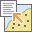The Summarize Within tool calculates statistics in areas where an input layer is within or overlaps a boundary layer. The area you are summarizing within can be an area layer or a hexagonal or square bin.

## Workflow diagram## Examples

• In order to complete routine maintenance projects efficiently, the city uses Summarize Within to count the street lights and to sum the miles of bike lanes within each maintenance assessment district. It can then estimate the material and staff needed to complete the work in each district.

• A cable provider is starting a pilot program where it provides low-cost Internet access to low-income community college students. Summarize Within can be used to determine the number of low-income families in each college district so the cable provider can choose an appropriate district for its pilot program.

• A development company is looking to create a new mixed-use project development in an urban center for the county. Within each city, the square area of potential development sites with good access to shops, restaurants, and light rail can be calculated using Summarize Within. This simplifies the site selection process.

## Usage notes

The inputs for Summarize Within include two layers. The first layer is the area used as a boundary to summarize your second layer, this is called the Summary Area and can be composed of an area layer you specify or square or hexagonal bins. The second layer specified is a point, line or area layer what will be summarized. This is called the Summarized Layer.

##### Tip:

Depending on the configuration of your organization, you will have access to either Esri Living Atlas Analysis Layers, such as counties and hexbins, or Custom Analysis Layers. Click the drop-down arrow for the Choose area layer to summarize other features within its boundaries parameter to select an analysis layer to use as a boundary.

A Count of Points, Total Length, or Total Area box will appear depending on the type of features to summarize in your layer. The boxes are checked by default and can only be unchecked if statistics are being calculated. The default distance measure will depend on the units in your profile.

TotalInput featuresDefaultOptions

Count of Points

Points

None

None

Total Length

Lines

Miles (U.S. Standard setting) or Kilometers (Metric setting)

• Miles
• Feet
• Kilometers
• Meters
• Yards

Total Area

Areas

Square Miles (U.S. Standard setting) or Square Kilometers (Metric setting)

• Square Miles
• Square Kilometers
• Square Meters
• Hectares
• Acres

You can optionally calculate standard statistics. For lines and areas, all weighted statistics will be calculated. Both the standard summary field statistics and the weighted summary field statistics are applied to data for the features in the Summarized Layer that intersect the Summary Area layer. The weighted summary field statistics are multiplied by a weight based on the proportion of the Summary Area intersecting each feature in the Summarized Layer. For standard statistics, there are eight options: count, sum, mean, minimum, maximum, range, standard deviation, and variance. There are two options for string statistics: count and any. There are six weighted statistics that are calculated on numeric fields in the layer to be summarized: count, sum, mean, minimum, maximum, and range. Weighted statistics are not calculated for string data. Each time a Field and Statistic are entered, a new row is added to the tool pane so more than one statistic can be calculated. View the summarized results in the result layer's table or pop-ups. By default, the count of features intersecting the Summary Area is always calculated.

It is important to consider the statistic you are calculating and what the data represents when choosing between standard and weighted statistics. For example, you may want to use weighted statistics for counts and amounts and standard statistics for rates and indices.

If Use current map extent is checked, only those features in the input layer and the layer to be summarized that are visible within the current map extent will be analyzed. If unchecked, all features in both the input layer and the layer to be summarized will be analyzed, even if they are outside the current map extent.

At Portal for ArcGIS 10.5.1, analysis using binning (hexagon or square) with a geographic projected coordinate system specified will automatically be projected to the coordinate system World Equal Area Cylindrical (wkid 54034) for analysis. To learn more about setting your coordinate system for analysis see: Use the analysis environments for GeoAnalytics Tools in the portal map viewer.

At 10.5.1 statistical calculations are computed using geodesic distances. At 10.5 calculations are computed using planar distances.

## Limitations

• Only summary areas that intersect at least one feature in the layer that is summarized will be included in the results.

## How Summarize Within works

### Equations

For summarized line and area features, weighted statistics incorporate Summary Area weights. None of the statistics for point features are weighted. The following table shows the equations used to calculate variance and the weighted mean.

StatisticEquationVariablesFeatures

Variance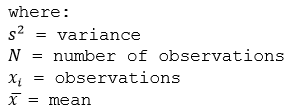Points

Weighted Mean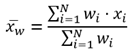Weights are calculated as the percentage of the feature i within the summary area.

Lines and Areas

### Points

Point layers are summarized using only the point features that fall within the Summary Area. Weighted statistics cannot be applied when summarizing points.

The figure and table below explain the statistical calculations of a point Summarized Layer within hypothetical areas. The Population field was used to calculate the statistics (Count, Sum, Minimum, Maximum, Range, Mean, Standard Deviation, and Variance) for the layer.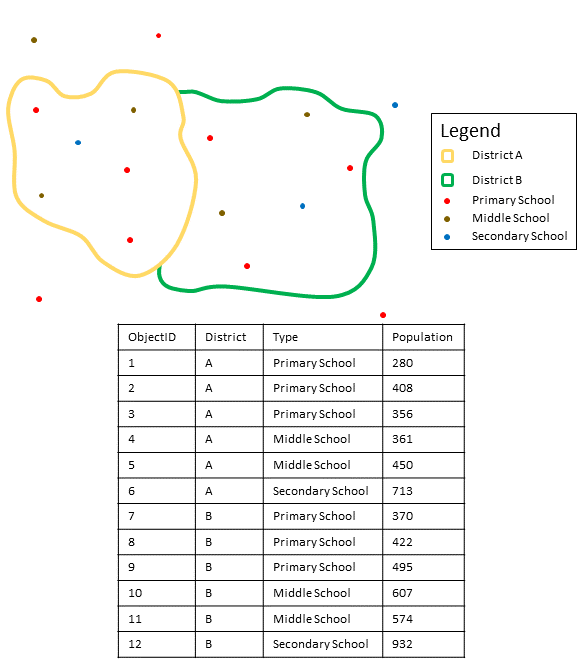Point layers are summarized using only points located within the area layer. An example attribute table is displayed above with values to be used in hypothetical statistic calculations.
Numeric statisticResults District A

Count

Count of:

``[280, 408, 356, 361, 450, 713] = 6``

Sum

``280 + 408 + 356 + 361 + 450 + 713 = 2,568``

Minimum

Minimum of:

``[280, 408, 356, 361, 450, 713] = 280``

Maximum

Maximum of:

``[280, 408, 356, 361, 450, 713] = 713``

Range

``713 - 280 = 433``

Mean

``2568/6 = 428``

Variance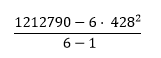``= 22737.2``

Standard Deviation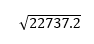``= 150.7886``
String statisticResults District A

Count

``= 6``

Any

= Secondary School

##### Note:

The count statistic (for strings and numeric fields) counts the number of nonnull values. The counts of [0, 1, 10, 5, null, 6] = 5. The count of [Primary, Primary, Secondary, null] = 3.

A real-life scenario in which this analysis could be used is in determining the total number of students in each school district. Each point represents a school. The Type field gives the type of school (elementary, middle school, or secondary) and a student population field gives the number of students enrolled at each school. The calculations and results are given for District A in the table above. From the results, you can see that District A has 2,568 students. When running the Summarize Within tool, the results would also be given for District B.

### Lines

For weighted statistics, line layers are summarized using only the proportions of line features that are within the Summary Area. Standard (non-weighted) statistics summarize any line intersecting the Summary Area. When summarizing lines using weighted statistics, use counts and amounts (rather than rates or indices) so proportional calculations make logical sense in your analysis.

The figure and table below explain the statistical calculations of a line Summarized Layer within a hypothetical Summary Area. The Volume field was used to calculate the statistics (Count, Sum, Minimum, Maximum, Range, Mean, Standard Deviation, and Variance) for the layer. The standard statistics are calculated using lines that intersect the boundary and the weighted statistics are calculated using the proportion of the lines that are within the Summary Area. Standard Deviation and Variance are only available for standard statistics.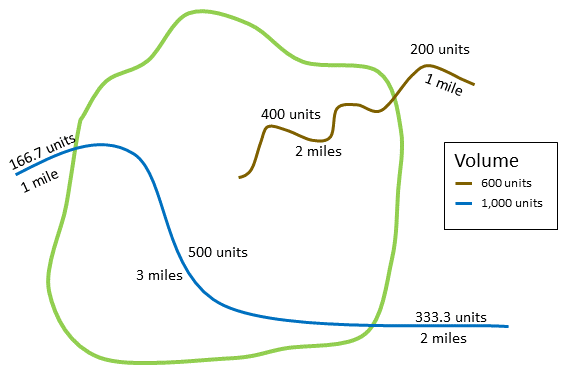Line layers are summarized using standard statistics and weighted statistics as shown below
Numeric statisticsStandard statisticsWeighted statistics

Calculating Weights

Weight of the brown line (value = 600):

``2/3 = .3333``

Weight of the blue line (value = 1000):

``3/6 = .5``

Count

Count of:

``[1000, 600] = 2``

Count of:

``1 x (3/6) + 1 x (2/3) = 1.667``

Sum

``1000 + 600 = 1600``
``1000 x (3/6) + 600 x (2/3) = 900``

Minimum

Minimum of:

``[1000, 600] = 600``

Minimum of:

``[1000 x (3/6), 600 x (2/3)]``
``[500, 400] = 400``

Maximum

Maximum of:

``[1000, 600] = 1000``

Maximum of:

``[1000 x (3/6), 600 x (2/3)]``
``[500, 400] = 500``

Range

``1600 - 600 = 1000``
``500 - 400 = 100``

Mean

``(1000 + 600)/2 = 800``
``(1000 x (3/6) + 600 x (2/3))/(3/6 + 2/3) ``
``(500 + 400)/(4/6) = 1350``

Variance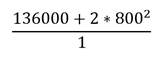``= 80000``

Not applicable

Standard Deviation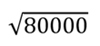``= 282.8427``

Not applicable

A real-life scenario in which this analysis could be used is in determining the total volume of water in rivers within the boundaries of a state park. Each line represents a river that is partially located inside the park. From the results, you can see that there are 5 miles of rivers within the park and the total volume is 900 units.

### Areas

Area layers are summarized using only the proportions of the area features that are within the input boundary. When summarizing areas, use fields with absolute numbers so proportional calculations make logical sense in your analysis. The results layer will be displayed using graduated colors.

Weighted statistics for summarized area layers are based on the proportions of the Summary Area features that are within the Summarized Layer. When summarizing areas, use counts or amounts (rather than rates or indices) so proportional calculations make logical sense in your analysis.

The figure and table below explain the statistical calculations of an area layer within a hypothetical Summary Area. The population field was used to calculate the statistics (Count, Sum, Minimum, Maximum, Range, Mean, Standard Deviation, and Variance) for the layer. The standard statistics are calculated using areas that intersect the Summary Area, and the weighted statistics are calculated using a proportional weight based on the portion of summary areas contained within each Summarized Layer. Standard Deviation and Variance are only available for standard statistics.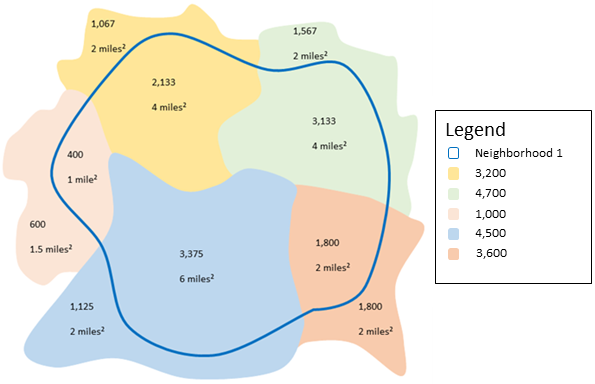Summary statistics are computed for areas in the Summarized Layer that intersect the summary areas. Weights for weighted statistics are based on the proportion of the summary areas that overlap the summarized layer features.
Numeric statisticsStandard statistics: Results Neighborhood 1Weighted statistics: Results Neighborhood 1

Calculating Weights

Weight of the yellow area (value = 3200):

``4/(2+4) = 3/4``

Weight of the green area (value = 4700):

``4/(2+4) = 2/3``

Weight of the pink area (value = 1000):

``1/(1+1.5) = 2/5``

Weight of the blue area (value = 4500):

``6/(2+6) = 3/4``

Weight of the orange area (value = 3600):

``2/(2+2) = 1/2``

Count

Count of:

``[3200, 4700, 1000, 4500, 3600] = 5``

Count of:

``(3/4)+(2/3)+ (2/5)+(3/4)+ (1/2) = 3.06667``

Sum

``3200 + 4700 + 1000 + 4500 + 3600 = 17000``
``(3/4) x 3200 +(2/3) x 4700 + (2/5) x 1000 +(3/4) x 4500 + (1/2) x 3600 = 11108.33``

Minimum

Minimum of:

``[3200, 4700, 1000, 4500, 3600] = 1000``

Minimum of:

``[(3/4) x 3200, (2/3) x 4700, (2/5) x 1000, (3/4) x 4500, (1/2) x 3600]``
``[2400, 3133.33, 400, 3375, 1800] = 400``

Maximum

Maximum of:

``3200, 4700, 1000, 4500, 3600] = 4700``

Maximum of:

``[2400, 3133.33, 400, 3375, 1800] = 3375``

Range

``4700 - 1000 = 3700``
``3,375 - 400 = 2,975``

Mean

``(17000)/5 = 3400``
``(11108.33)/[3.06667] = 3622.83``

Variance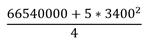``= 2185000``

Not applicable

Standard Deviation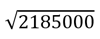``= 1478.175``

Not applicable

## Similar tools

Use Summarize Within to calculate statistics for features that overlap a boundary layer. Other tools may be useful in solving similar but slightly different problems.

### Map viewer analysis tools

If you are trying to summarize points and want to apply time stepping, use the Aggregate Points tool.

### ArcGIS Desktop analysis tools

This GeoAnalytics Tools is available in ArcGIS Pro.

Summarize Within performs the functions of the Spatial Join and Summary Statistics tools.